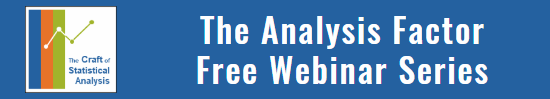# 6 Types of Dependent Variables that will Never Meet the Linear Model Normality Assumption

by

The assumptions of normality and constant variance in a linear model (both OLS regression and ANOVA) are quite robust to departures.  That means that even if the assumptions aren’t met perfectly, the resulting p-values will still be reasonable estimates.

But you need to check the assumptions anyway, because some departures are so far that the p-value become inaccurate.  And in many cases there are remedial measures you can take to turn non-normal residuals into normal ones.

But sometimes you can’t.

Sometimes it’s because the dependent variable just isn’t appropriate for a linear model.  The dependent variable, Y, doesn’t have to be normal for the residuals to be normal (since Y is affected by the X’s).

The errors do.

But the distribution of the errors is related to the distribution of Y. So Y does have to be continuous, unbounded, and measured on an interval or ratio scale.

If you go through the Steps to Statistical Modeling, Step 3 is: Choose the variables for answering your research questions and determine their level of measurement. Part of the reason for doing this is to save yourself from running a linear model on a DV that just isn’t appropriate and will never meet assumptions.

Some of these include DVs that are:

If you have one of these, Stop.  Do not pass Go.  Do not run a linear model.

Hopefully you noticed this at Step 3, not when you’re checking assumptions, which is Step 11.

But luckily, there are other types of regression procedures available for all of these variables.Binary, Ordinal, and Multinomial Logistic Regression for Categorical Outcomes
Get beyond the frustration of learning odds ratios, logit link functions, and proportional odds assumptions on your own. See the incredible usefulness of logistic regression and categorical data analysis in this one-hour training.

### Related PostsAlberto

Hi I have recently completed a log regression of 1 categorical variable vs 4 dependent variables. I have found the z score and chi values for these regressions however now I would like to know how i could rank the values within these variables to find “confidence intervals” ie if the value of the dependant variable is above X value what is the confident that this will cause the categorical variable to be “yes” or “no” for example.
Thanks
Albertoalen owen

How can i change non-normal data into normal data in order to be suitable for GLM?Mark

Hello. I would like to run a regression where the independent variable is continuous but values cannot be greater than 1 or less than -1. I also have six categroical variables with 3 levels each. What sort of regression can I run for this?

Thanks MarkAnees Khan

Kindly help, Is there any Normality assumption required for RATIO and Dummy Independent Variable? I m confused

thanks

AneesKaren

Hi Anees,

There are no distributional assumptions for Independent Variables in a regression. See this: https://www.theanalysisfactor.com/the-distribution-of-independent-variables-in-regression-models-2/jenny

Help! I’m currently trying to run a 2x2x2x2 mixed factorial anova with 4 IVs and accuracy/success rates (described as %) in SPSS. My data is anything but normally distributed but I also don’t know which transformation to use to make it better. Any ideas would be so much appreciated!Peter Flom

Nice post.

“Unbounded” is interesting. if the bounds are very far from the mean (in standardized terms) it can be OK. Take, for example, weight of human adults. This has a lower bound. It certainly can’t be less than 0! Yet that’s fine, because that is so far from the mean.Karen

Thanks, Peter.

I agree. I think it’s not even that the bound is so far from the mean, but even that it’s so far from any data points. The practical problem is when you get ceiling and floor effects–when a lot of observations are butted up against the bound.

It’s similar to the idea of using a linear regression instead of logistic, when all the probabilities are in the middle (say between .2 and .8). Because the sigmoidal logistic regression function is linear in the middle, you’ll get pretty much the same results. It’s close to 1 and 0 (the bounds) where logistic regression can accommodate the fact that the relationship isn’t linear.

Karen